# Convert Any Unit

The Fastest Way To Convert Units
Paid Version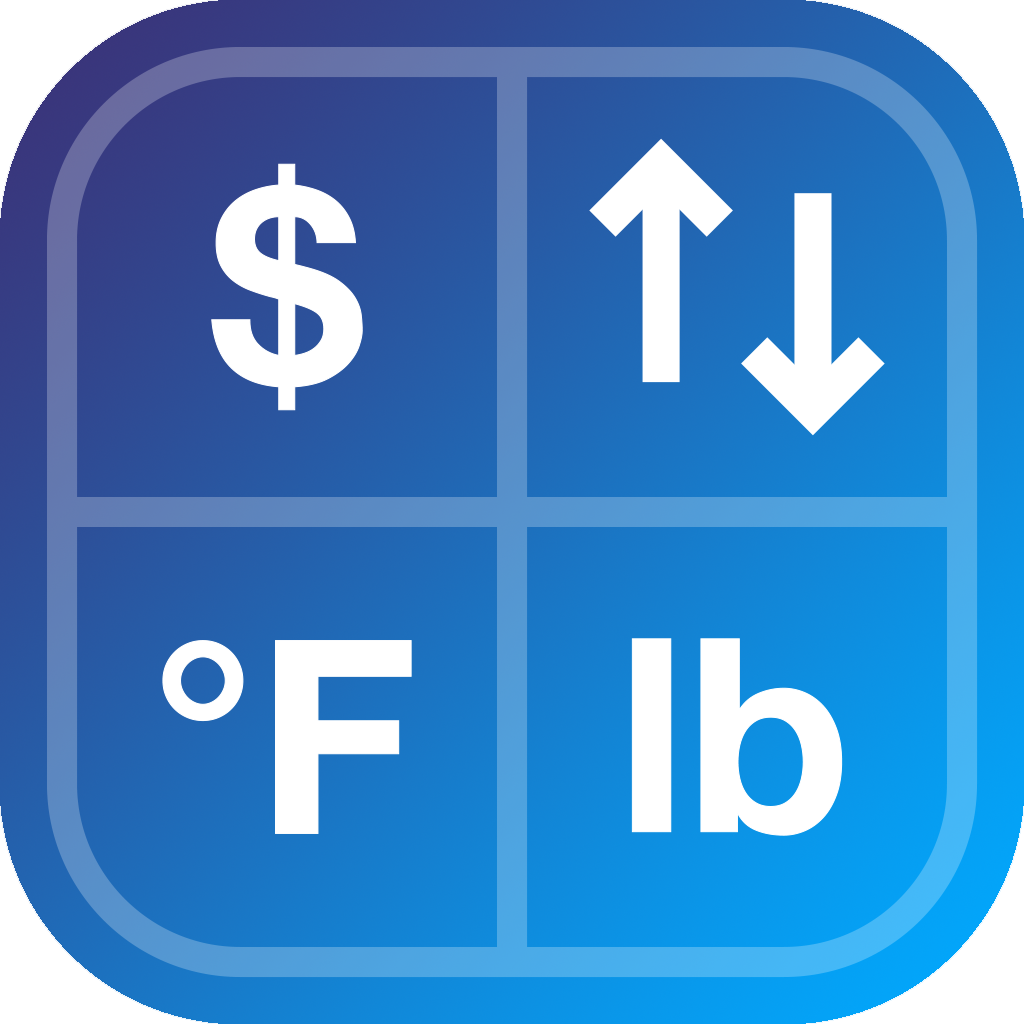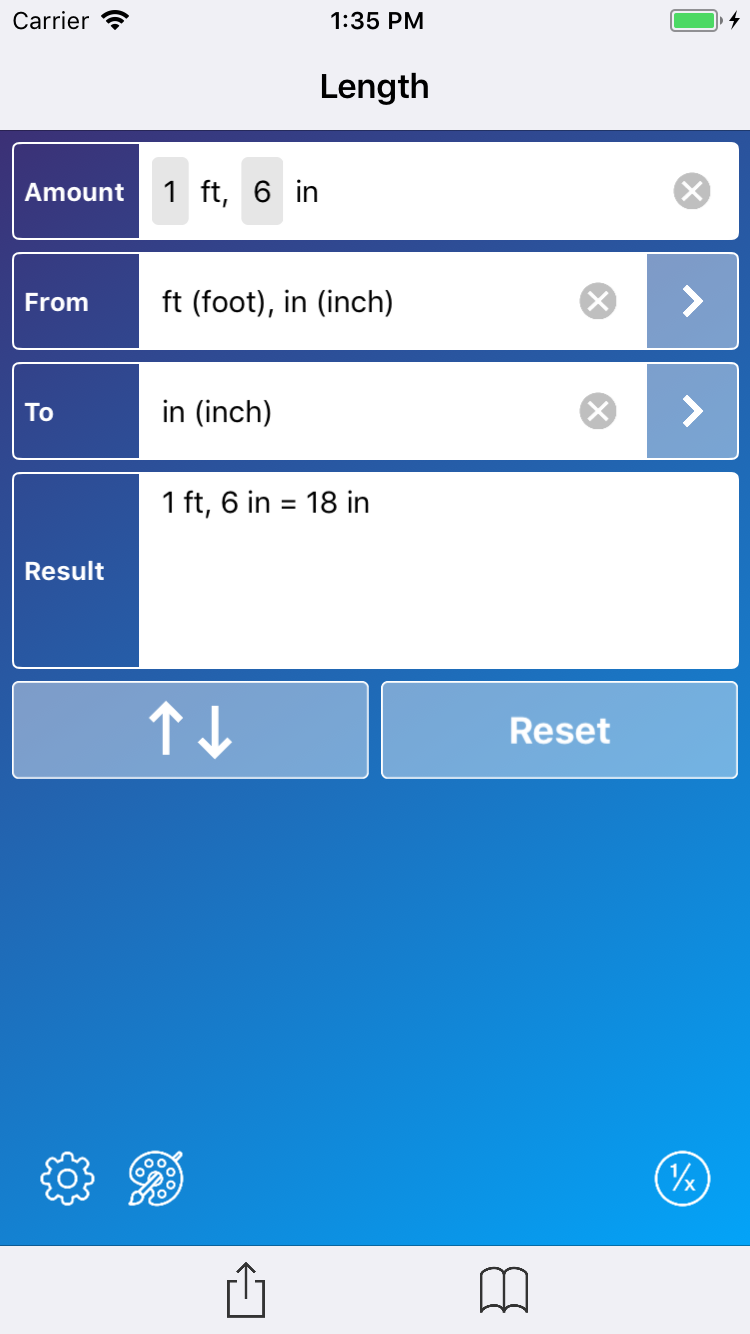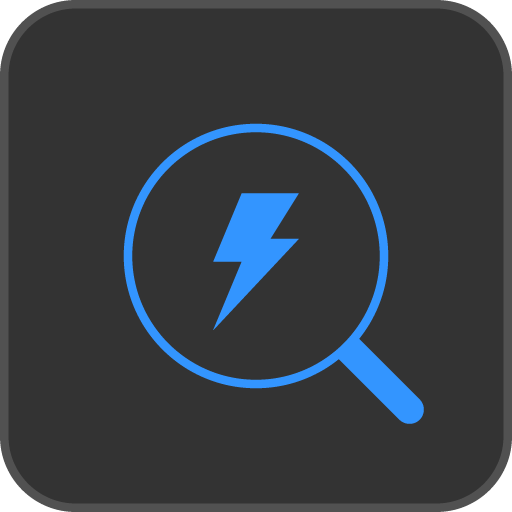## Super Fast

• Just type in a unit without first selecting the category. As you type, a list of most likely units appear. Tap a unit to select it. For most units, 1 or 2 letters is enough to find the unit you want.
• The category of the To unit is automatically restricted to the category of the From unit.
• As you search for the second unit, the conversion is automatically shown in line with the search results.
• To see how fast it is to select units, watch this video: https://www.youtube.com/watch?v=GJMH9kdj2v4&feature=youtu.be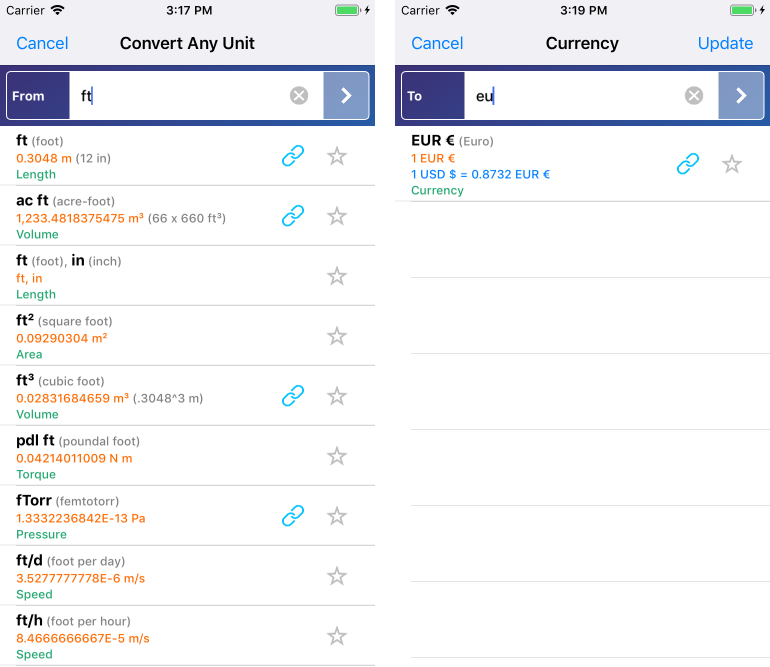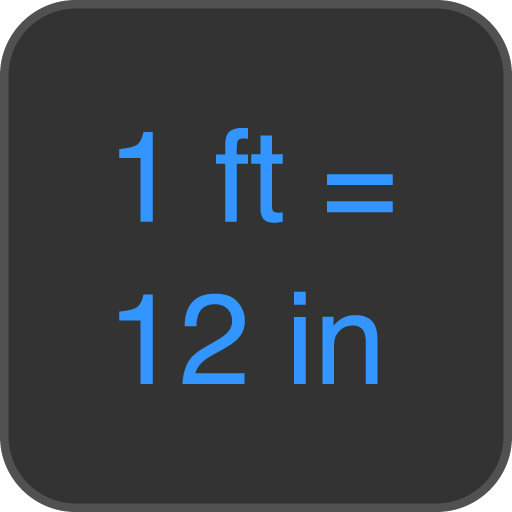## Easy to Understand

• The result of the unit conversion is shown as an easy to understand equation.
• The formula for converting between units is shown below the conversion result.
• Both unit symbols and names are shown.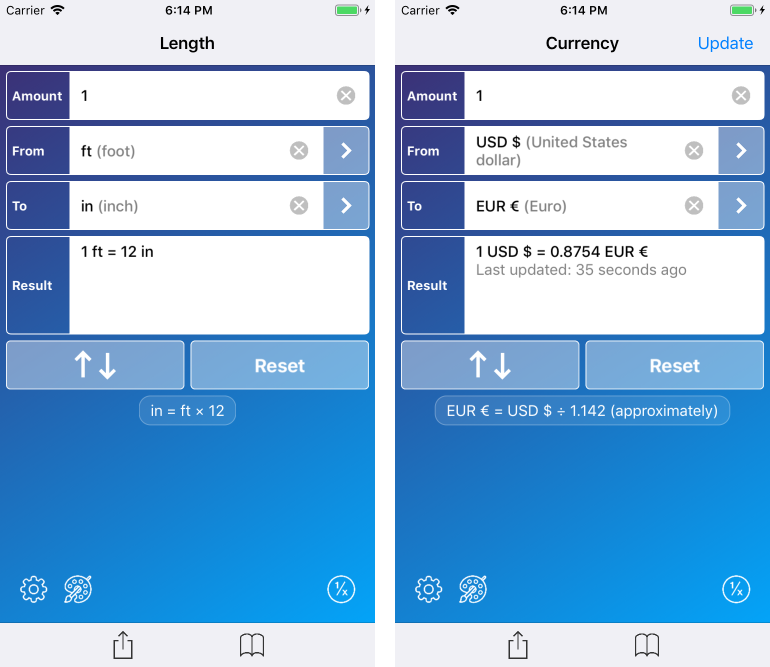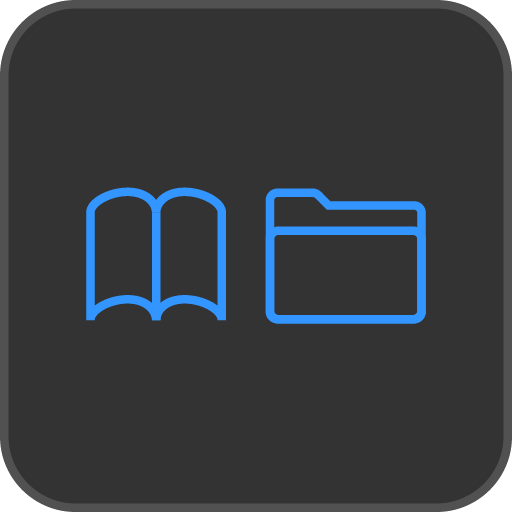## Bookmarks

• Save bookmarks of your favorite conversions.
• Drag and drop to reorder them and organize them into folders.
• Currency units conversions are shown with the current exchange rate.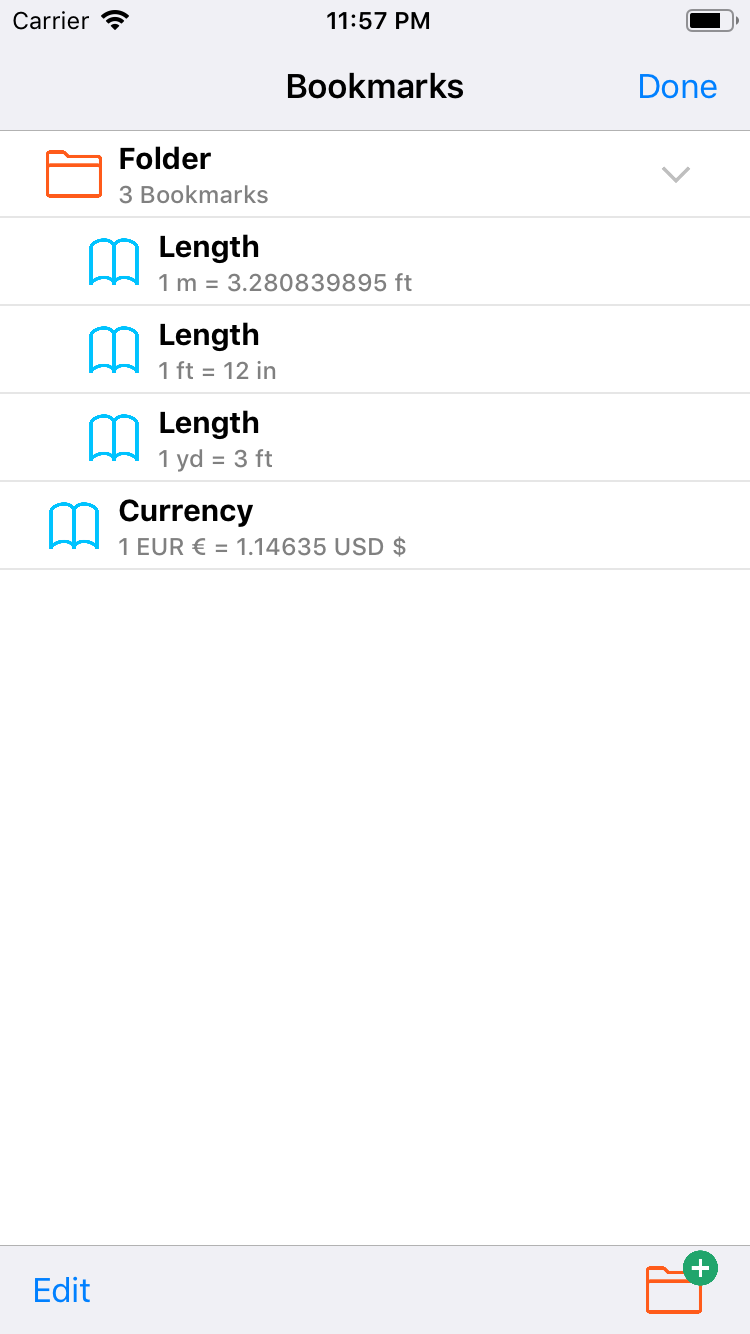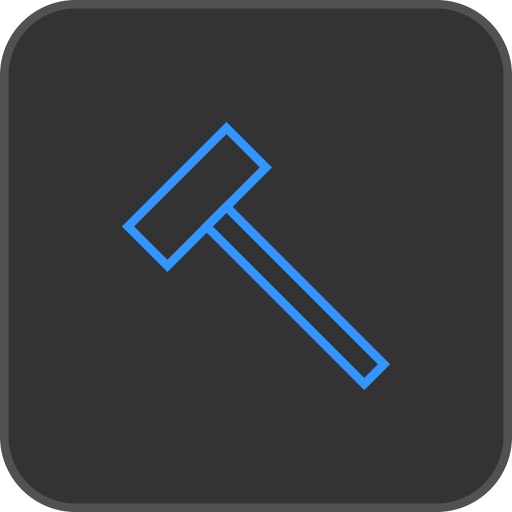## Custom Units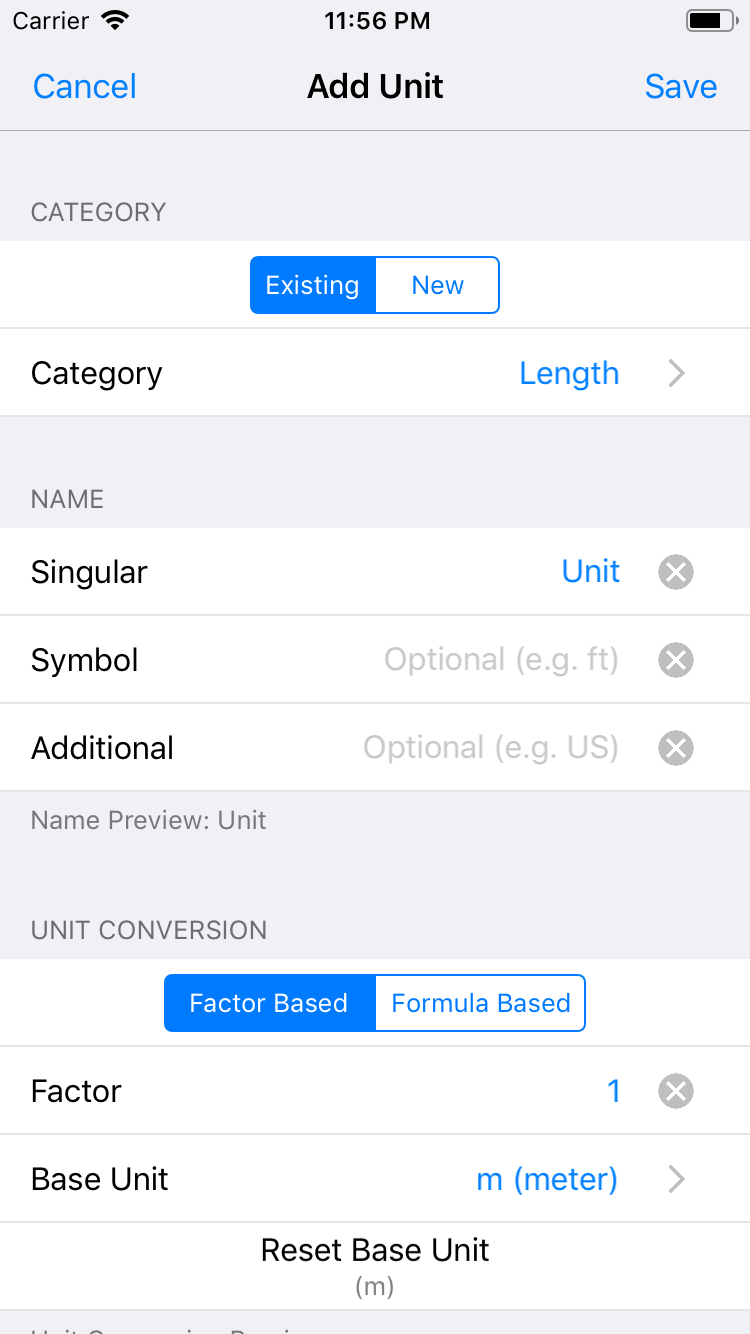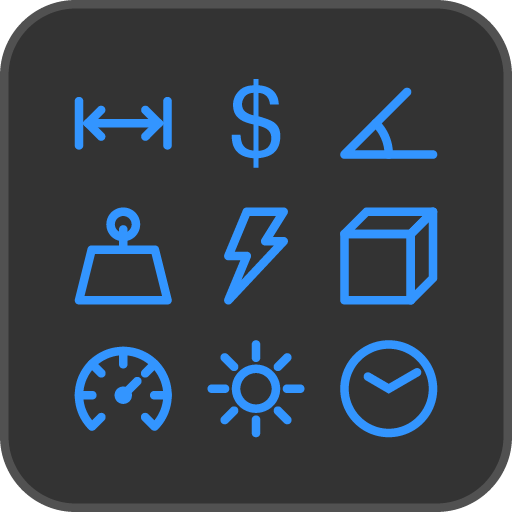## Over 6,400 Units In 51 Categories

• Acceleration
• Angle
• Area
• Blood Cholesterol
• Blood Glucose
• Capacitance
• Currency
• Currency / Volume
• Data
• Data Rate
• Density
• Dynamic Viscosity
• Electric Charge
• Electric Current
• Electric Potential
• Electrical Conductance
• Electrical Resistance
• Energy
• Force
• Frequency
• Fuel Economy
• Illuminance
• Inductance
• Kinematic Viscosity
• Length
• Luminance
• Magnetic Field Intensity
• Magnetic Flux
• Magnetic Flux Density
• Mass
• Mass Flow
• Mass Fraction
• Mass Moment of Inertia
• Mole Fraction
• Numeral Systems
• Pace (Speed)
• Power
• Pressure
• Radiation Rate - Equivalent Dose
• Sound
• Speed
• Temperature
• Time
• Torque
• Volume
• Volume Flow
• Volume / Area
• Water Hardness
• Weight (Mass)
• Wire Gauge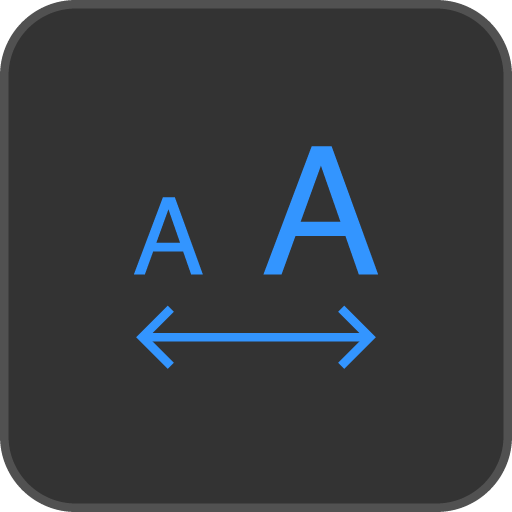• Adjust the font size in 5% increments.
• Increase up to 140% bigger.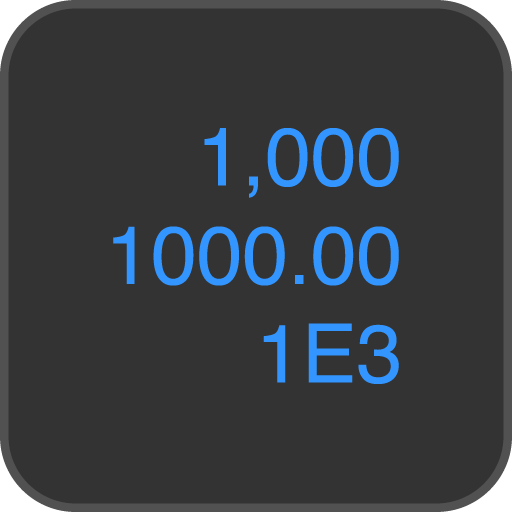## Automatic Number Formatting

• Thousands Separators.
• Adjustable minimum / maximum Decimal Digits.
• Scientific or Engineering E Notation.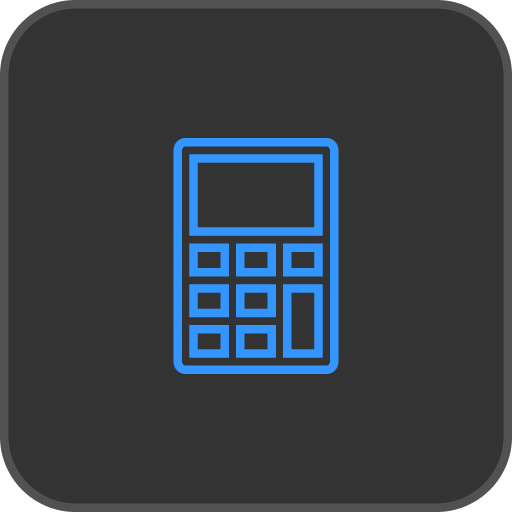## Inline Calculator

• Calculate an equation as your input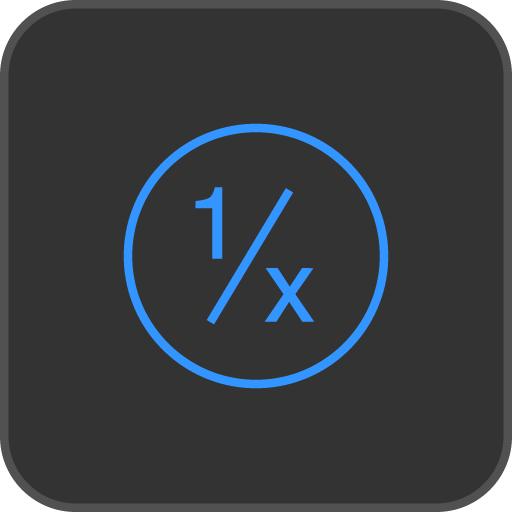## Convert To Fraction

• A clever Convert to Fraction slider, lets you quickly convert the unit conversion to the nearest fraction
• The fraction result is stated as being Exact or Rounded to the nearest fraction.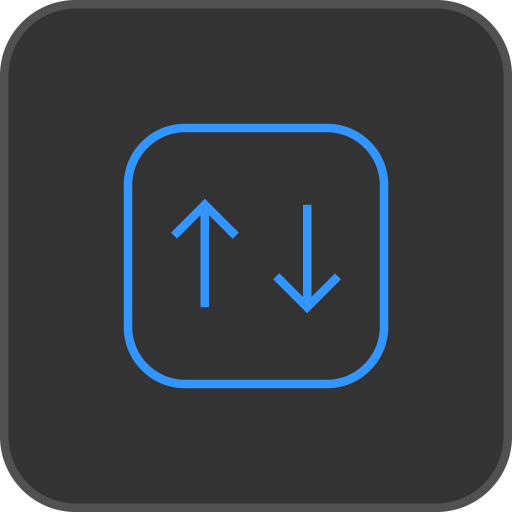## Flip Units Button

• Quickly switch the From and To units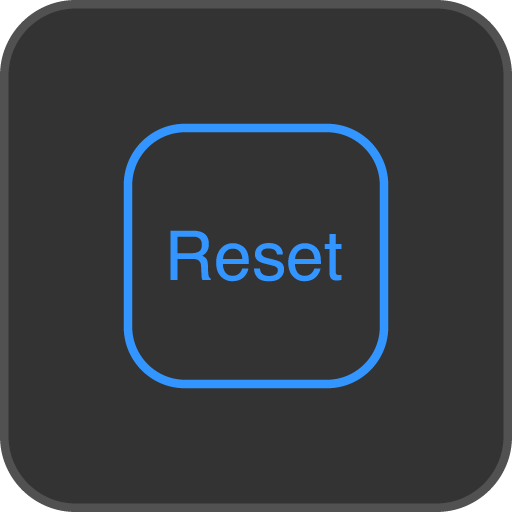## Reset Button

• Quickly clear all inputs and start over.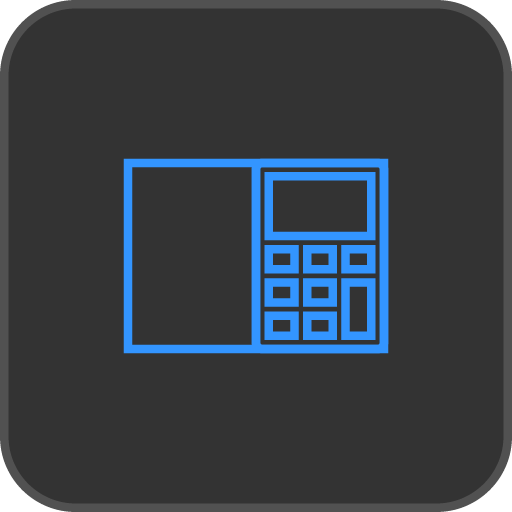## Split View

• On the iPad, use the app at the same time as other apps.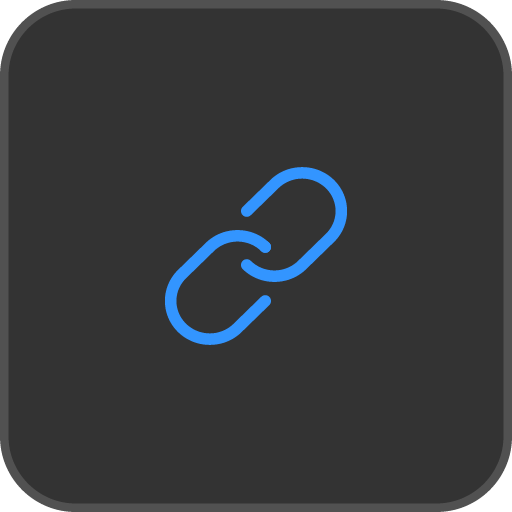## URL Scheme

• convertanyunit://
• convertanyunitfree://